# Arrays in Excel Formula

In this article, we will learn how to use arrays in excel formula.

What is an Array?
An array is a set of data elements. It is just a list or range of values e.g. {1,2,3} or {“Apples”, ”kiwi”, “lemon”}

Let’s understand Array usage using it an example.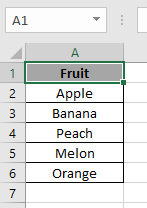We will use OR function operating arrays. As you know OR function in Excel runs logic_test. So arrays of values will result in arrays of result.

Use the formula

=OR (A2:A6 = "Apple")

A2:A6 array: {“apple”, “Banana”, “Peach”, “Melon”, “Orange”}
Resultant array: {True, False, False, False, False}
the OR function returns TRUE If any element in the resultant array is TRUE as shown below in the snapshot.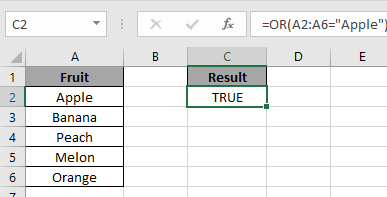Now we will operate two arrays side by side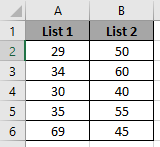We need to find the maximum difference between the corresponding values e.g. B2-C2 = 50-29 = 21 & B4-C4 = 40-30 = 10

So use the formula

{=MAX(B2:B6-A2:A6)}

Use Ctrl + shift + Enter to get corresponding results so as to operate corresponding values.

Note: Do not enter the curly braces manually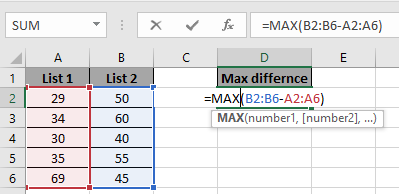Array{ 50-29 , 60-34 , 40-30 , 55-35 , 69-45}
Resultant array{ 21, 26, 10 , 20 , 24}
Result{ 26 }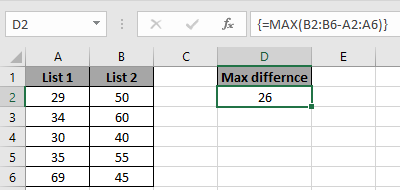Hope you understood how to use Arrays in Excel formula. Explore more articles on Excel function here. Please feel free to state your query or feedback for the above article.

Related Articles

How to Return An Array Using in Excel Using INDEX Function in Excel

How to use INDEX and MATCH function to Lookup Value in Excel

How to use the LOOKUP function in Excel

How to Lookup Value with Multiple Criteria in Excel

Popular Articles:

50 Excel Shortcuts to Increase Your Productivity

How to use the VLOOKUP Function in Excel

How to use the COUNTIF function in Excel

How to use the SUMIF Function in Excel

Terms and Conditions of use

The applications/code on this site are distributed as is and without warranties or liability. In no event shall the owner of the copyrights, or the authors of the applications/code be liable for any loss of profit, any problems or any damage resulting from the use or evaluation of the applications/code.